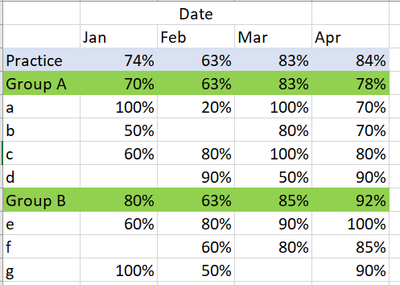cancel
Showing results for
Did you mean:Frequent Visitor

## Issues with having a right formula

I am trying to get the right subtotals and total averages in the utilisation matrix report I am creating. I am using this DAX: IFERROR(IF(ISINSCOPE('X'[Y]),[sum]/[count],[Utilisation]),BLANK())

I have different type of utilisation, and for some reason the results that it provide in both are the same and I am not sure why the "sum" formula does not provide the right infromation I need.

sum = SUMX(Table, Utilisation)
#Thank you
5 REPLIES 5Frequent Visitor

So I have Practice on top, then group and the resource, divided by months. But my matrix has some blanks on the resources, so I want that the subtotals for Group and Practice has the right average of utilisation ignoring the blanks.

Something like this:Is that something you have reviewed in the past?Super User

``````using Utilisation_Product =
AVERAGEX ( VALUES ( 'X'[Y] ), COALESCE ( [Utilisation], 0 ) )``````Super User

Hi @alexacg

if Utilisation is a measure tye

sum = SUMX ( VALUES ( 'X'[Y] ), [Utilisation] )Frequent Visitor

Hi Tamerj1, thank you so much for your help on this :). That definitely solved my sum formula, but it did not fix my error on the matrix to average correctly the product and subproduct categories I have ignoring the blanks, and I was using Utilisation_Product= IFERROR(IF(ISINSCOPE('X'[Y]),[sum]/[count],[Utilisation]),BLANK()), any idea about this?Super User

How does your matrix look like? What columns are placed in the matrix? What results are you expecting?